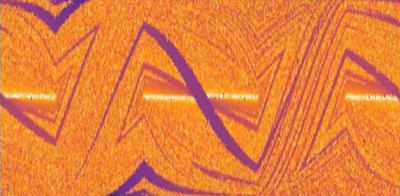# Research in Probability, Analysis and DynamicsProbability, Analysis and Dynamics are intricately intertwined branches of mathematics. The problems raised and investigated in either of them is mostly inspired by common interests in mathematics and other sciences. All three research groups consist of outstanding and internationally leading scientists ranging from mid- to established career stages, accompanied by energetic and enthusiastic groups of early career colleagues.

Research on various aspects of Probability Theory, the science of uncertainty around us, leads to frontier-line results concerning applications of information-theoretic methods to prove fundamental theorems in probability, and limit theorems on stochastic processes that originate from statistical physics, biology, computer science or combinatorial structures like random graphs and networks, scaling limits of interacting particle systems, processes with long memory.

Mathematical Analysis is the precise treatment of calculus, the study of change. A main strand of research in the Analysis group is the interplay between the spectrum of the Laplace operator with self-adjoint boundary conditions and the geometry of the manifold or domain in Euclidean space, closely related to topics such as stochastic analysis of Brownian motion, and tools such as the theory of function spaces, calculus of variations and shape optimization techniques. Other research themes in the group include geometric function theory, which is concerned with how infinitesimal properties of functions, such as conformality, have large-scale geometric consequences.

Ergodic Theory is the branch of mathematics that investigates the “chaotic” properties of Dynamical Systems and has provided powerful tools to solve some outstanding problems in other fields such as in number theory, combinatorics, quantum chaos and statistical physics. We carry out several strands of research in these fields, such as homogeneous dynamics, Teichmüller dynamics, dimension theory and fractal analysis, mathematical billiards, flows on surfaces and Diophantine approximation.